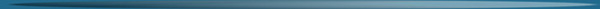# Lesson 6: For, while, if, and relationsLike most computer languages, MATLAB offers a variety of flow control statements like for, while and if. The statements that we use to control the flow are called relations.

## For statement

For example, for a given n, the statement
```	x = []; for i =1:n, x=[x,i^2], end
```
or
```	x = [];
for i = 1:n
x = [x,i^2]
end
```
will produced a certain n-vector and the statement
```	x = []; for i = n:-1:1, x=[x,i^2], end
```
will produced the same vector in reverse order. Try them. Note that a matrix may be empty (such as x = []). The statements
```	for i = 1:m
for j = 1:n
H(i,j) = 1/(i+j-1);
end
end
H
```
will produced and print to the screen the m × n hilbert matrix. The semicolon on the inner statement suppresses printing of unwanted intermediate results while the last H displays the final result.

## While statement

The general form of a while loop is
while relation
statements
end

The statements will be repeatedly executed as long as the relation remains true. For example, for a given number a, the following will compute and display the smallest nonnegative integer n such that 2 to the power of n is > a:

```	n = 0;
while 2^n <= a
n = n + 1;
end
n
```

## If statement

The general form of a simple if statement is
if relation
statements
end
The statements will be executed only if the relation is true. Multiple branching is also possible, as illustrated by
```	if n < 0
parity = 0;
elseif rem(n,2) == 0
parity = 2;
else
parity = 1
end
```
In two-way branching the elseif portion would be omitted.

## Relations

The relational operators in MATLAB are
```	<	less than
>	greater than
<=	less than or equal
>=	greater than or equal
==	equal
~=	not equal
```

Note that "=" is used in an assigment statement while "==" is used in a relation. Relations may be connected or quantified by the logical operators:

```	&	and
|	or
~	not
```
When applied to scalars, a relation is actually the scalar 1 or 0 depending on whether the relation is true or false. Try 3 < 5, 3 > 5, 3 == 5 and 3 == 3. When applied to matrices of the same size, a relation is a matrix of 0's and 1's giving the value of the relation between corresponding entries. Try a = rand(5), b = triu(a), a == b.

A relation between matrices is interpreted by while and if to be true if each entry of the relation matrix is nonzero. Hence, if you wish to execute statement when matrices A and B are equal, you could type

if A == B
statement
end
but if you wish to execute statement when A and B are not equal, you would type
if any(any(A ~= B))
statement
end
or more simply,
if A == B else
statement
end
Note that the seemingly obvious
if A ~= B, statement, end
will not give what is intended since statement would be executed only if each of the corresponding entries of A and B differ. The functions any and all can be creatively used to reduced matrix relations to vectors or scalars. Two any's are required above since any is a vector operator.

The for statement permits any matrix to be used instead of 1:n. See the User's Guide for details of how this feature expands the power of the for statement.